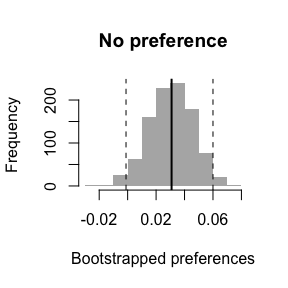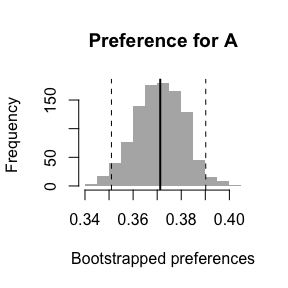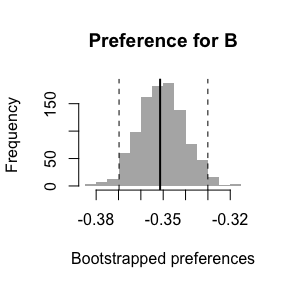# Estimating Preference For Ranking Functions With Clicks On Interleaved Search Results

## Introduction

The way Wikimedia Search Platform’s (formerly Wikimedia Discovery) analyses have been assessing changes to search has traditionally relied on A/B testing wherein the control group receives results using the latest configuration and the test group (or groups) receives results using the experimental configuration. Another way to evaluate the user-perceived relevance of search results from the experimental configuration relies on a technique called interleaving. In it, each user is their own baseline – we perform two searches behind the scenes and then interleave them together into a single set of results using the team draft algorithm described by Chapelle et al. (2012):

1. Input: result sets $$A$$ and $$B$$.
2. Initialize: an empty interleaved result sets $$I$$ and drafts $$T_A, T_B$$ for keeping track of which results belong to which team.
3. For each round of picking:
1. Randomly decide whether we first pick from $$A$$ or from $$B$$.
2. Without loss of generality, if $$A$$ is randomly chosen to go first, grab top result $$a \in A$$, append it to $$I$$ and $$T_A$$: $$I \gets a, T_A \gets a$$.
3. Take the top result $$b \in B$$ such that $$b \neq a$$ and append it to $$I$$ after $$a$$ and to $$T_B$$: $$I \gets b, T_B \gets b$$.
4. Update $$A = A \setminus \{a, b\}$$ and $$B \setminus \{a, b\}$$ so the two results that were just appended to $$I$$ are not considered again.
5. Stop when $$|I| = \text{maximum per page}$$, so only the first page contains interleaved results.
4. Output: interleaved results $$I$$ and team drafts $$T_A, T_B$$.

By keeping track of which results belong to which ranking function when the user clicks on them, we can estimate a preference for one ranker over the other. The preference statistic $$\Delta_{AB}$$ is described by Chapelle et al. as

$\Delta_{AB} = \frac{\text{wins}_A + \frac{1}{2} \text{ties}}{\text{wins}_A + \text{wins}_B + \text{ties}} - 0.5,$

where wins are calculated by counting clicks on the results from teams “A” and “B”. A positive value of $$\Delta_{AB}$$ indicates that $$A \succ B$$, a negative value indicates that $$B \succ A$$. We performed two types of calculations: per-session and per-search. In per-session, “A” has won if there are more clicks on team “A” results than team “B” results and $$\text{wins}_A$$ is incremented by one for each such session. In per-search, “A” has won if there are more clicks on team “A” results in each search, thus any one session can contribute multiple points to the overall $$\text{wins}_A$$.

In order to obtain confidence intervals for the preference statistic, we utilize bootstrapping with $$m$$ iterations.

1. For bootstrap iteration $$i = 1, \ldots, m$$:
1. Sample unique IDs with replacement.
2. Calculate $$\Delta_{AB}^{(i)}$$ from new data.
2. The confidence intervals (CIs) are calculated by finding percentiles of the distribution of bootstrapped preferences $$\{\Delta_{AB}^{(1)}, \ldots, \Delta_{AB}^{(m)}\}$$ – e.g. the 2.5th and 97.5th percentiles for a 95% CI.

## Simulated Data

This package provides simulated search and click data. The three built-in datasets have simulated users that (1) exhibit no preference, (2) exhibit preference for the ranking function “A”, and (3) exhibit preference for the ranking function “B”.

data(interleaved_data, package = "wmfastr") # no preference
data(interleaved_data_a, package = "wmfastr") # preference for A
data(interleaved_data_b, package = "wmfastr") # preference for B

Here are the first few rows of the third dataset:

knitr::kable(head(interleaved_data_b))
session_id timestamp event position ranking_function
ufxl7jprs8 2018-08-01 00:01:06 serp NA NA
zbuk6t3n4m 2018-08-01 00:00:37 serp NA NA
zbuk6t3n4m 2018-08-01 00:03:16 click 8 B
zbuk6t3n4m 2018-08-01 00:03:37 click 13 B
zbuk6t3n4m 2018-08-01 00:05:26 click 16 B
zbuk6t3n4m 2018-08-01 00:05:42 click 18 B

## Estimation

library(wmfastr)

To calculate $$\Delta_{AB}$$ with interleaved_preference, we will need to use the clicks. We also use bootstrapping via interleaved_bootstraps which resamples sessions (with replacement) to obtain a distribution of the preference statistic $$\Delta_{AB}$$. After we plot each bootstrapped sample, we mark the 95% confidence interval bounds. Note that interleaved_confint outputs the quantile-based CI and uses the same bootstrap function internally.

### No preference

When users click on the interleaved results without a preference, the resulting preference statistic is close to 0 and the confidence interval covers 0:

x <- interleaved_data[interleaved_data$event == "click", ] x <- x[order(x$session_id, x$timestamp), ] boot_x <- interleaved_bootstraps(x$session_id, x$ranking_function) hist(boot_x, col = "gray70", border = NA, main = "No preference", xlab = "Bootstrapped preferences") abline(v = quantile(boot_x, c(0.025, 0.975)), lty = "dashed") abline(v = interleaved_preference(x$session_id, x$ranking_function), lwd = 2)### Preference for A When users click on the interleaved results with a preference for A, the resulting preference statistic is positive and the confidence interval does not cover 0: y <- interleaved_data_a[interleaved_data_a$event == "click", ]
y <- y[order(y$session_id, y$timestamp), ]
boot_y <- interleaved_bootstraps(y$session_id, y$ranking_function)
hist(boot_y, col = "gray70", border = NA, main = "Preference for A", xlab = "Bootstrapped preferences")
abline(v = quantile(boot_y, c(0.025, 0.975)), lty = "dashed")
abline(v = interleaved_preference(y$session_id, y$ranking_function), lwd = 2)### Preference for B

When users click on the interleaved results with a preference for B, the resulting preference statistic is negative and the confidence interval does not cover 0:

z <- interleaved_data_b[interleaved_data_b$event == "click", ] z <- z[order(z$session_id, z$timestamp), ] boot_z <- interleaved_bootstraps(z$session_id, z$ranking_function) hist(boot_z, col = "gray70", border = NA, main = "Preference for B", xlab = "Bootstrapped preferences") abline(v = quantile(boot_z, c(0.025, 0.975)), lty = "dashed") abline(v = interleaved_preference(z$session_id, z\$ranking_function), lwd = 2)